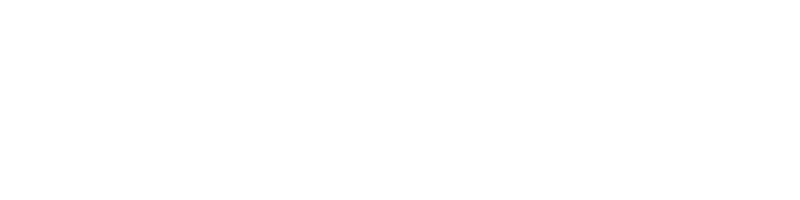# 4. ЦиклПри построении цикла

• определить количество повторений
• выделить изменяемые параметры
Построение окружностей
``````
x = 40

for i in range(5):
draw.circle(window, (x, 40), 20)
x += 100
``````Вложение цикла в цикл

• выделить внешний и внутренний цикл
• определить количество повторений циклов
• выделить изменяемые параметры
Построение окружностей
``````
x = 40
y=100
for k in range (2)
for i in range(5):
draw.circle(window, (x, y), 20)
x += 100
y +=60
``````
Задачи